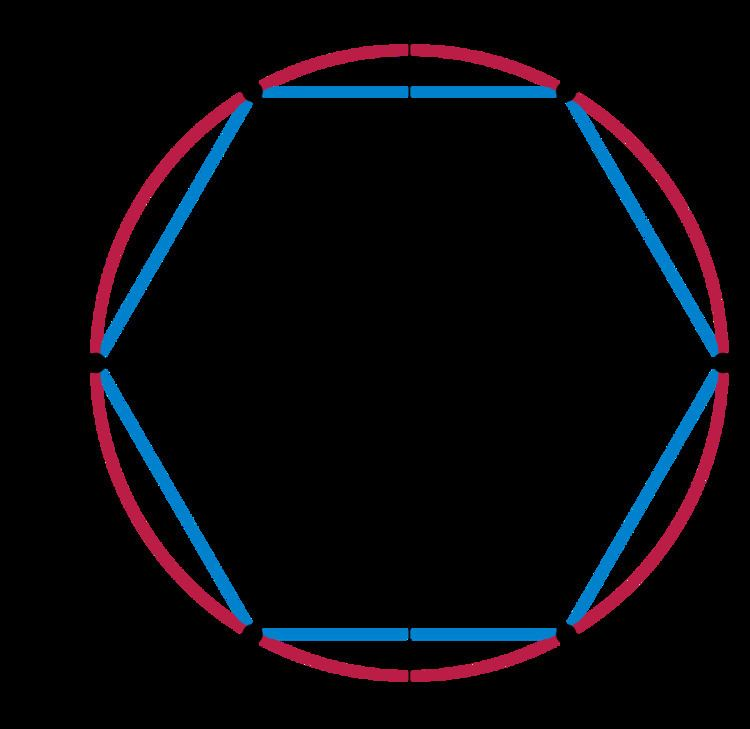# Algebraic group

Updated on
Covid-19In algebraic geometry, an algebraic group (or group variety) is a group that is an algebraic variety, such that the multiplication and inversion operations are given by regular maps on the variety.

## Contents

In terms of category theory, an algebraic group is a group object in the category of algebraic varieties.

## Classes

Several important classes of groups are algebraic groups, including:

• Finite groups
• GL(n, C), the general linear group of invertible matrices over C
• Jet group
• Elliptic curves.
• Two important classes of algebraic groups arise, that for the most part are studied separately: abelian varieties (the 'projective' theory) and linear algebraic groups (the 'affine' theory). There are certainly examples that are neither one nor the other — these occur for example in the modern theory of integrals of the second and third kinds such as the Weierstrass zeta function, or the theory of generalized Jacobians. But according to Chevalley's structure theorem any algebraic group is an extension of an abelian variety by a linear algebraic group. This is a result of Claude Chevalley: if K is a perfect field, and G an algebraic group over K, there exists a unique normal closed subgroup H in G, such that H is a linear group and G/H an abelian variety.

According to another basic theorem, any group in the category of affine varieties has a faithful finite-dimensional linear representation: we can consider it to be a matrix group over K, defined by polynomials over K and with matrix multiplication as the group operation. For that reason a concept of affine algebraic group is redundant over a field — we may as well use a very concrete definition. Note that this means that algebraic group is narrower than Lie group, when working over the field of real numbers: there are examples such as the universal cover of the 2×2 special linear group that are Lie groups, but have no faithful linear representation. A more obvious difference between the two concepts arises because the identity component of an affine algebraic group G is necessarily of finite index in G.

When one wants to work over a base ring R (commutative), there is the group scheme concept: that is, a group object in the category of schemes over R. Affine group scheme is the concept dual to a type of Hopf algebra. There is quite a refined theory of group schemes, that enters for example in the contemporary theory of abelian varieties.

## Algebraic subgroup

An algebraic subgroup of an algebraic group is a Zariski-closed subgroup. Generally these are taken to be connected (or irreducible as a variety) as well.

Another way of expressing the condition is as a subgroup which is also a subvariety.

This may also be generalized by allowing schemes in place of varieties. The main effect of this in practice, apart from allowing subgroups in which the connected component is of finite index > 1, is to admit non-reduced schemes, in characteristic p.

## Coxeter groups

There are a number of analogous results between algebraic groups and Coxeter groups – for instance, the number of elements of the symmetric group is n ! , and the number of elements of the general linear group over a finite field is the q-factorial [ n ] q ! ; thus the symmetric group behaves as though it were a linear group over "the field with one element". This is formalized by the field with one element, which considers Coxeter groups to be simple algebraic groups over the field with one element.

## Glossary of algebraic groups

There are a number of mathematical notions to study and classify algebraic groups.

In the sequel, G denotes an algebraic group over a field k.

## References

Similar Topics
Valmiki (2009 film)
Harendra Singh Malik
Lowell Palmer
Topics

 B i Link H2
 L